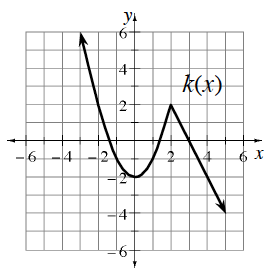### Home > PC3 > Chapter 4 > Lesson 4.2.3 > Problem4-101

4-101.​ Throughout this book, key problems have been selected as “checkpoints.” Each checkpoint problem is marked with an icon like the one at left. These checkpoint problems are provided so that you can check to be sure you are building the skills from this course at the expected level. If you have trouble with checkpoint problems, refer to the review materials and practice problems that are available in the Checkpoint Materials at cpm.org.

This problem is a checkpoint for graphing transformations of functions.Given the graph of $y=k(x)$ at right, sketch the following transformations.

1. $y=k(x-2)+3$

2. $y=k(-x)-2$

3. $y=\frac{1}{2}k(x)+1$